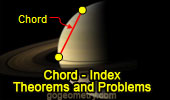# Online Plane Geometry: Chord of a Circle, Theorems and Problems

 Circle Chord, Theorems and Problems 1Euclid's Elements Book I, 23 Definitions. One-page visual illustration. Euclid's Elements Book. Index Common Chord to two circles. Index. Theorems and Problems. Perpendicular Chords. Index. Theorems and Problems. Parallel Chords. Index. Theorems and Problems. Eyeball Theorem: Animated Angle to Geometry Study. Equal chords. Mascheroni construction with compass alone. Index Common chords. Euclid's Elements, Book XIII, Proposition 10 One page visual illustration. Simson Line. A proof of Simson line with animation. Miquel's Pentagram with Dynamic Geometry. You can alter the pentagram dynamically in order to test and prove (or disproved) conjectures and gain mathematical insight that is less readily available with static drawings by hand. Requires Java Plug-in 1.3 or higher. Please be patient while the applet loads on your computer. If you are using a dial-up connection, it may take a few minutes but is well worth the wait. Cabri, GSP, Cinderella, C.a.R. Four Circles Theorem Using Interactive Dynamic Software Step-by-Step construction, Manipulation, and animation. Common chords Three Circles Theorem Using TracenPoche Dynamic Software Common chords. Butterfly Theorem Proof with animation. Midpoint of a chord. Ptolemy's Theorem. Chords Mascheroni construction: Find the center of a circle with compass alone. Common chords. Intersecting Circles Index. Common chords. Cyclic Quadrilateral. Chords Brahmagupta's Formula  Area of a cyclic quadrilateral. Go to Page: Previous | 1 | 2 | 3 | 4 | 5 | 6 | 7 | 8 | 9 | 10 | Next

 Home | Geometry | Circle | Email | Post a comment | By Antonio Gutierrez Last updated: Dec 5, 2017